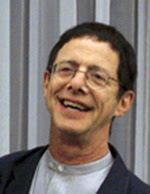## Tuesday, December 11, 2007

### The Solution to the Problem of Induction

Yes, Leonard Peikoff has the answer to this centuries-old problem. And it can be yours too for just US\$205 and 13hrs of your time."These historic lectures present, for the first time, the solution to the problem of induction—and thereby complete, in every essential respect, the validation of reason."- ARI blurb

Excellent!Anonymous said...

The write-up for this course indicates that, "The course concludes that (apart from mathematics) the same process of induction is essential to every rational field"

This seems funny to me, as mathematics is one of the few (perhaps only) domain where inductive methods exist and are logically proven. Why the parenthetical "(apart from mathematics)"??????

Does his theory of induction ignore mathematics because its the easy case, or (more likely, IMO) because this course is yet another example of objectivist verbiage and hand waving that fails to deliver when applied to real concrete examples?

JayCross said...

hahahahahahahahaha

I'm sorry. I like Leonard and absolutely love OPAR but that is the best picture you could've chosen for this post.

Ellen Stuttle said...

Mathematical induction is a special (and possibly confusingly named) case because it's a form of deduction.

See, e.g.:

http://www.earlham.edu/~peters/courses/logsys/math-ind.htm

"Mathematical Induction,"
by Peter Suber, Philosophy Department, Earlham College:

"In ordinary induction, we examine a certain number of particular cases and then generalize.
[....]

"Strong inductions differ from valid deductions in one important way: in the induction, the conclusion contains information that was not contained in the premises. This is the source of the uncertainty of inductions. (Conversely, deductions are certain because they tautologously restate their premises.) Inductions are strengthened as confirming instances pile up. But they can never bring certainty unless every possible case is actually examined, in which case they become deductions. [....]

"'Mathematical induction' is unfortunately named, for it is unambiguously a form of deduction. However, it has certain similarities to induction which very likely inspired its name. It is like induction in that it generalizes to a whole class from a smaller sample. In fact, the sample is usually a sample of
one, and the class is usually infinite. Mathematical induction is deductive, however, because the sample plus a rule about the unexamined cases actually gives us information about every member of the class. Hence the conclusion of a mathematical induction does not contain more information than was latent in the premises. Mathematical inductions therefore conclude with deductive certainty."

Here's a site describing the technique of mathematical induction:

http://www.themathpage.com/aPreCalc/mathematical-induction.htm

It expresses the principle of mathematical induction as:

"If

1) when a statement is true for the natural number n = k,
then it is also true for its successor, n = k + 1;

and

2) the statement is true for n = 1;

then the statement is true for every natural number n.
"

EllenAnonymous said...

If you liked that picture, you'll love his pirate costume on youtube.Anonymous said...

Ellen,
Confusing terminology can be gotten around by just calling Peikoff's historic solution to the problem of induction, "LPINDUCTION" and then applying it mathematical problems. Why cannot LPINDUCTION be applied to the domain of mathematics?

If his solution is so historic, applying to to math problems would be a great way to demonostrate and teach how it works.

(BTW, thanks for the good quotes and link)

Ellen Stuttle said...

anonymous said:

"Why cannot LPINDUCTION be applied to the domain of mathematics?

If his solution is so historic, applying to to math problems would be a great way to demonostrate and teach how it works.
"

Anon, although you thanked me for the links and quotes I provided, it seems you didn't understand their point: "mathematical induction" ISN'T "induction"; it's deduction. Hence whatever Leonard thinks he's accomplished in regard to the "historic problem of induction," it wouldn't apply to mathematical induction, which never has been an instance of that problem.

EllenAnonymous said...

I take back my thanks